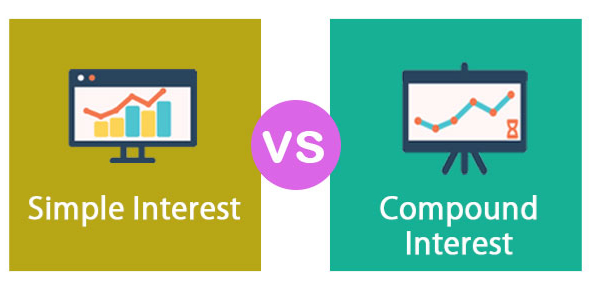# SSC CGL Exam Tier-I: Simple And Compound Interest Quiz!

20 Questions | Attempts: 1005
ShareSettingsQuiz Description

• 1.
A sum of money placed at compound interest doubles itself in 4 yr. In how many years will it amount to four times itself?
• A.

12 yr

• B.

13 yr

• C.

8 yr

• D.

16 yr

• 2.
The difference between the compound interest and simple interest on Rs. 10000 for 2 yr is Rs. 25. The rate of interest per annum is
• A.

5%

• B.

7%

• C.

10%

• D.

12%

• 3.
The simple interest on a sum of money is  of the principal and the number of years is equal to the rate percent per annum. The rate percent per annum is equal to
• A.

3%

• B.
• C.
• D.
• 4.
At some rate of simple interest, A lent Rs. 6000 to B for 2 yr and Rs. 1500 to C for 4 yr and received Rs. 900 as interest from both of them together. The rate of interest per annum was
• A.

5%

• B.

6%

• C.

8%

• D.

10%

• 5.
What annual payment will discharge a debt of Rs. 6450 due in 4 yr at 5% per annum simple interest?
• A.

Rs. 1400

• B.

Rs. 1500

• C.

Rs. 1550

• D.

Rs. 1600

• 6.
In how many years will a sum of money double itself at simple interest per annum?
• A.

24 yr

• B.

20 yr

• C.

16 yr

• D.

12 yr

• 7.
At what time will Rs. 10000 amount to Rs. 13310 at 20% per annum compounded half-yearly?
• A.
• B.

2 yr

• C.
• D.

3 yr

• 8.
In what time Rs. 8000 will amount to Rs. 9261 at 10% per annum compound interest, when the interest is compounded half-yearly?
• A.
• B.
• C.
• D.
• 9.
A certain sum amounts to Rs. 5832 in 2 yr at 8% per annum compound interest, then the sum is
• A.

Rs. 5000

• B.

Rs. 5200

• C.

Rs. 5280

• D.

Rs. 5400

• 10.
In how many years will a sum of Rs. 800 at 10% per annum compound interest, compounded semi-annually becomes Rs. 926.10?
• A.
• B.
• C.
• D.
• 11.
Simple interest on Rs. 500 for 4 yr at 6.25% per annum is equal to the simple interest on Rs. 400 at 5% per annum for a certain period of time. The period of time is
• A.

4 yr

• B.

5 yr

• C.
• D.
• 12.
If the difference between the compound and simple interests on a certain sum of money for 3 yr at 5% per annum is Rs. 15.25, then the sum is
• A.

Rs. 2000

• B.

Rs. 1000

• C.

Rs. 1500

• D.

Rs. 2500

• 13.
If the simple interest for 6 yr be equal to 30% of the principal, it will be equal to the principal after
• A.

20 yr

• B.

30 yr

• C.

10 yr

• D.

22 yr

• 14.
The effective annual rate of interest, corresponding to a nominal rate of 6% per annum payable half-yearly is
• A.

6.06%

• B.

6.07%

• C.

6.08%

• D.

6.09%

• 15.
What annual installment will discharge a debt of Rs? 6450 due in 4 yr at 5% simple interest?
• A.

Rs. 1500

• B.

Rs. 1835

• C.

Rs. 1935

• D.

Rs. 1950

• 16.
The difference between the simple and compound interest on a certain sum of money for 2 yr at 4% per annum is Rs. 4. The sum is
• A.

Rs. 2500

• B.

Rs. 2400

• C.

Rs. 2600

• D.

Rs. 2000

• 17.
The compound interest on a certain sum of money at a certain rate for 2 yr is Rs. 40.80 and the simple interest on the same sum is Rs. 40 at the same rate and for the same time. The rate of interest per annum is
• A.

2%

• B.

3%

• C.

4%

• D.

5%

• 18.
A sum of money becomes eight times of itself in 3 yr at compound interest. The rate of interest per annum is
• A.

100%

• B.

80%

• C.

20%

• D.

10%

• 19.
At what rate per cent per annum will the simple interest on a sum of money be  of the amount in 10 yr?
• A.
• B.
• C.
• D.
• 20.
The ratio of the principal and the amount after 1 yr is 10: 12. Then, the rate of interest per annum is
• A.

12%

• B.

16%

• C.

18%

• D.

20%

## Related TopicsBack to top
×

Wait!
Here's an interesting quiz for you.